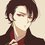# This came in my exam today

Q) Write the expression $-2x^2 - 6x + 15$ in the form of $a ( x + p)^2 + q$ where a, p and q are integers.

Can anyone help me out.?Note by Syed Hamza Khalid
2 years, 4 months ago

This discussion board is a place to discuss our Daily Challenges and the math and science related to those challenges. Explanations are more than just a solution — they should explain the steps and thinking strategies that you used to obtain the solution. Comments should further the discussion of math and science.

When posting on Brilliant:

• Use the emojis to react to an explanation, whether you're congratulating a job well done , or just really confused .
• Ask specific questions about the challenge or the steps in somebody's explanation. Well-posed questions can add a lot to the discussion, but posting "I don't understand!" doesn't help anyone.
• Try to contribute something new to the discussion, whether it is an extension, generalization or other idea related to the challenge.

MarkdownAppears as
*italics* or _italics_ italics
**bold** or __bold__ bold
- bulleted- list
• bulleted
• list
1. numbered2. list
1. numbered
2. list
Note: you must add a full line of space before and after lists for them to show up correctly
paragraph 1paragraph 2

paragraph 1

paragraph 2

[example link](https://brilliant.org)example link
> This is a quote
This is a quote
    # I indented these lines
# 4 spaces, and now they show
# up as a code block.

print "hello world"
# I indented these lines
# 4 spaces, and now they show
# up as a code block.

print "hello world"
MathAppears as
Remember to wrap math in $$ ... $$ or $ ... $ to ensure proper formatting.
2 \times 3 $2 \times 3$
2^{34} $2^{34}$
a_{i-1} $a_{i-1}$
\frac{2}{3} $\frac{2}{3}$
\sqrt{2} $\sqrt{2}$
\sum_{i=1}^3 $\sum_{i=1}^3$
\sin \theta $\sin \theta$
\boxed{123} $\boxed{123}$

Sort by:

Here is my full solution :

\begin{aligned} a(x + p)^2 + q & = - 2x^2 - 6x + 15 \\ a(x^2 + 2px + p^2) + q & = -2x^2 - 6x + 15 \\ {\color{#3D99F6}a}x^2 + {\color{#E81990}2ap}x + {\color{#20A900} ap^2 + q} & = {\color{#3D99F6}-2}x^2 {\color{#E81990}-6}x + {\color{#20A900}15} \\ \end{aligned}

On comparing both the sides we get, $a = -2, p = \dfrac32, q = \dfrac{39}{2}$. So, the final result is

$-2x^2 - 6x + 15 = -2 \left( x - \dfrac32 \right)^2 + \dfrac{39}{2}$

@Syed Hamza Khalid, as you said there is no possibility for $p,q$ to be integers. So, the question must be having a typo or it wants to say rational numbers instead of integers.

- 2 years, 4 months ago

Yeah thats wat I think

- 2 years, 4 months ago

Even I don't know- I got $-2( x + \dfrac{3}{2} )^2 - \dfrac{21}{2}$ but the question asked $a, p$ and $q$ are integers.. and these are not $\therefore$ I think there is a typo error in the question perhaps. But how did u get $+ \dfrac{39}{2}$ at the end

- 2 years, 4 months ago

$-ap^2 + q = 15$ from which we will get q.

- 2 years, 4 months ago

I just used complete the square: $= -2x^2 - 6x +15 \\ = -2(x^2 + 3x) + 15 \\ = -2((x + \dfrac{3}{2})^2 - \dfrac{9}{4} ) - 15 \\ = -2(x + \dfrac{3}{2})^2 + \dfrac{9}{2} - 15 \\ = -2(x + \dfrac{3}{2} )^2 - \dfrac{21}{2} . \square$

This was the method we learned at school $\therefore$ I applied it but I don't understand why I didn't get integers

- 2 years, 4 months ago

I will check once again.

- 2 years, 4 months ago

Why did you write "-15" in the third step instead of "+15" ?

- 2 years, 4 months ago

I got it as $-2(x + \dfrac32)^2 + \dfrac{39}{2}$. Is it correct.

- 2 years, 4 months ago

Hah, what a question! Have you used a research paper service like https://ca.edubirdie.com/research-paper-writing-services to complete these tasks or not? I'm gonna pass my exams soon and want to know the answer.

- 1 year, 6 months ago